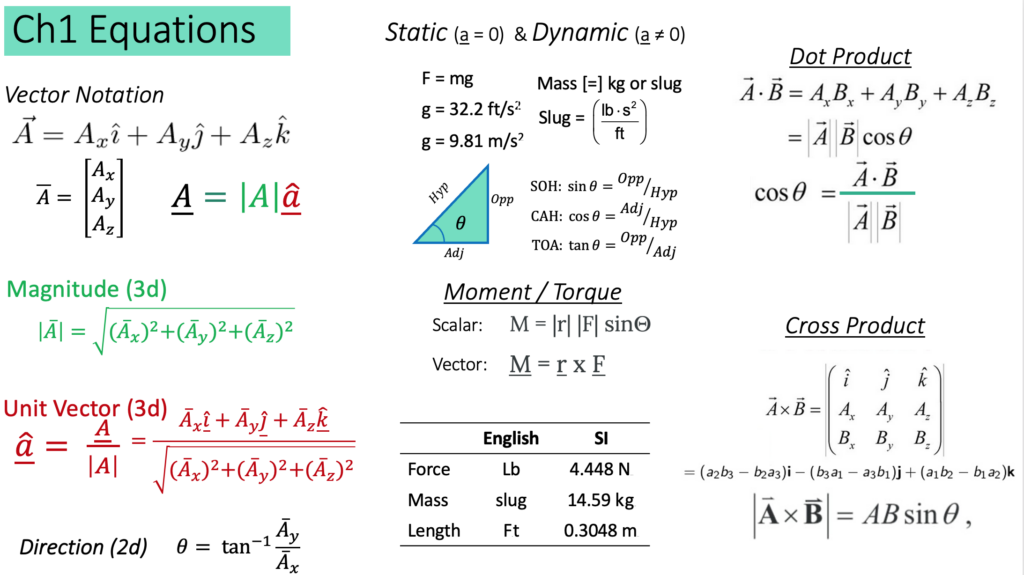# Chapter 1: Fundamental Concepts

Static vs Dynamic Motion

Before we start, what is the difference between static and dynamic? Static problems are all problems where there is no acceleration. As you’re driving down the road and you’re cruising along at a constant velocity, that is a static problem. As soon as you start to slow down for a stop light or speed up, you are in dynamic motion, and that’s much more complicated. For this course, we will only consider problems where there is no motion (such as the ‘static’ is used in the English language), or constant velocity. Be prepared to give many examples of static versus dynamic, because before you can solve a problem, you have to know what type of problem it is!

Introduction to Chapter 1: Fundamental Concepts

This chapter has a lot of concepts from math and physics that are necessary for you to understand before we can apply them in engineering contexts. It’s kind of like: before you can write an essay to express your opinion, you need to know how to write the a, b, c’s and what each word means. Here, you need to know how to compute a cross product before you can calculate how much Torque is created from a force.

Some of this might be new. Some of this might be familiar, but we might be applying it in a different way. (Such as calculating torque – this is not what you learned in high school physics!) Some of it might feel new, so practice practice practice!

Here are the sections in this Chapter:

Here are the key equations and concepts you will learn in this chapter